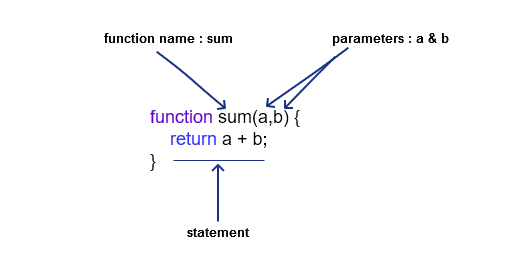# Functions In JavaScript

Share

A function in JavaScript is a re-usable block of code which is designed to be used any number of times once it is created. They do not get executed until invoked or called upon.

You can notice a function in JavaScript with a structure that features a pair of parenthesis – ( )

## A Basic Function

###### Function Declaration
function sum(a,b) {
return a + b;
}
###### Implementation

sum(2, 4);    // Output : 6

sum(5, 0);    // Output : 5

In the above example, we have a function named ‘sum‘ with a and b as its parameters. A function can have multiple parameters or no parameters at all. In this case, the function ‘sum‘ is returning the sum of a and b. By default functions return undefined.

## Function Declaration

There are several ways to define a function. The above example is just one of the many ways. This typical way of defining a function is called – function declaration or function statement.

Lets take a look at its composition :It consists of a function keyword followed by a name – ‘sum’ and a parentheses – ( ). The parentheses may include parameter names separated by commas. The number of parameters that a function can take actually depends on the JavaScript engine. In our case, we just have two – a and b.

• name

The name of the function.

• parameter

The arguments to be passed to the function.
Number of arguments varies in different JavaScript engines.

• statements

Comprise the body of the function.
Holds the script code to be executed by that function.

Function declarations in JavaScript are hoisted to the top of the enclosing function or global scope.

i.e : You can use the function before you declare it.

sum(2,4);  // Output : 6
function sum(a,b) {
return a + b;
}

## Function Expression

Another way of defining a function is by using a function expression.

In general Terms, Statements involving functions which do not start with function are function expressions.

Function Expression is very similar to the function declaration approach, except for the function name. In function expression you can neglect the name of the function. The function, hence created without a name is often called an anonymous function.

It is however possible to create functions in function expressions with a name. Providing a name for the function helps in error tracking as the stack trace will contain the name of the function which helps in finding the origin of the error.

###### Function Expression
var sum = function(a,b) {
return a + b;
}
###### Implementation

sum(2, 4);    // Output : 6

sum(5, 0);    // Output : 5

Function expressions in JavaScript are not hoisted unlike function declaration.
You can not use function expressions before you define them.

sum(2,4);  // TypeError: sum is not a function
function sum(a,b) {
return a + b;
}

Function expression can be used as an IIFE (Immediately Invoked Function Expression) which runs as soon as it is defined.

## Function Constructor

In cases where you need to create functions dynamically, you can call the Function constructor.

However, calling the constructor directly can lead to security and various performance issues.

var sum = new Function(‘a’, ‘b’, ‘return a + b’);

sum(2,4);  // Output : 6

Every JavaScript function is actually a Function object.

Functions created using Function constructor will only be able to access their own local variables and global ones, not the ones from the scope in which the constructor was created.

## Immediately Invoked Function Expressions

IIFE (Immediately Invoked Function Expression) is a function expression that runs as soon as it is defined. It is also referred to as Self-Executing Anonymous Function.

Style 1
(function() {
statements
})();
Style 2
(function() {
statements
}());
Style 3
!function() {
statements
}();

It is a design pattern which is often used in cases where the function needs to be called upon only once. It is applicable when you need to protect the scope of your function and the variables that it contains.

There are various styles for creating an IIFE. Style 1 and 2 shown in the above examples are mostly used. Style 3 is a different variation using a unary operator. Other supported operators are ‘+’, ‘-‘ and ‘~’.

Variables defined within the expression can not be accessed from outside it.

## Arrow Functions

An arrow function is a shorter syntax for writing a regular function expressions. It is a new feature added since ES6. Using it helps us avoid typing certain keywords like function and return.

Arrow functions do not have their own bindings for this, arguments, super or new.target.

###### Basic Syntax

(param1,…. paramN) => { statements }

singleParam => { statements }

params => ({ data : data2})  // return object literals

(param1, …rest) => { statements } // rest parameter

More about Arrow Functions will be provided in the Arrow Functions Deep Dive.

## Generator Functions

Generator Function is one of the new feature added since ES6. It introduces a new way of working with iterators and functions in the form of Generators.

###### Example

function* generator(i) {
yield i;
yield i + 10;
}

###### Implementation

var gen = generator(10);
console.log(gen.next().value);  // output: 10
console.log(gen.next().value);  // output: 20